> 文档中心 > xmind转Excel测试用例

# xmind转Excel测试用例

### xmind转Excel测试用例

• 一、引言
• 二、环境准备
• 三、脚本编写
• 1、读取xmind文件
• 2、根据读取数据，解析数据
• 3、定义测试用例标题
• 4、数据写入Excel中
• 5、运行入口
• 四、总结

# 二、环境准备

``python 3.7.1xmindparser 1.0.9openpyxl 3.0.9``# 三、脚本编写

## 1、读取xmind文件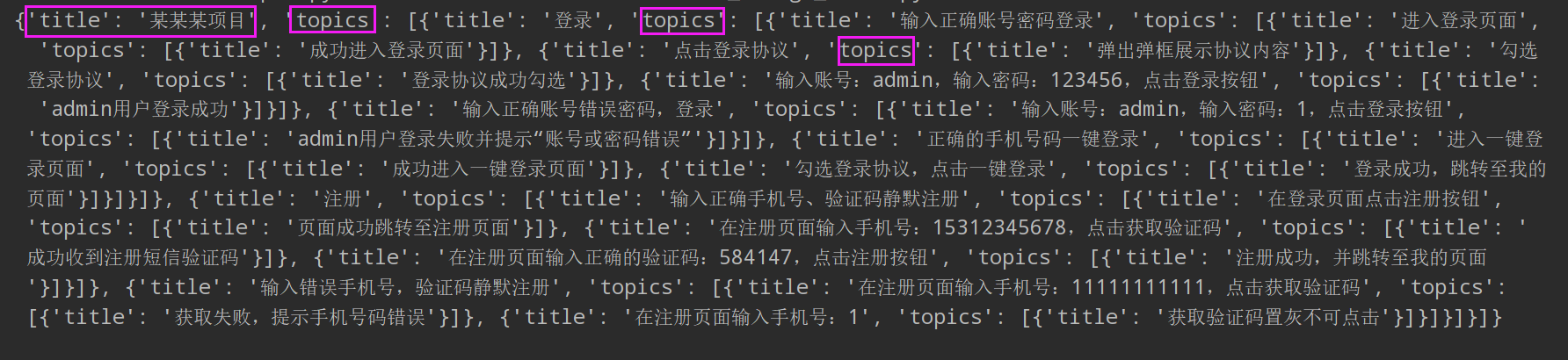``from xmindparser import xmind_to_dictdef read_xmind_data(xmind_file_path):    """    读取xmind文件，返回中心主题标题和其他分支list数据    :param xmind_file: xmind文件路径    :return:    """    case_data_dict=xmind_to_dict(xmind_file_path)["topic"]    title = case_data_dict["title"]    data_list=case_data_dict["topics"]    return title,data_list``

## 2、根据读取数据，解析数据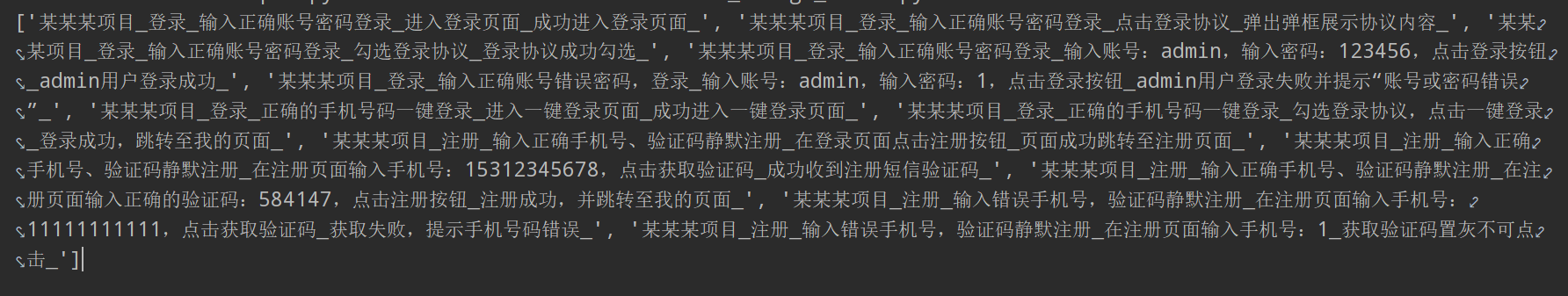``def xmind_to_caselist(data_list,title,listcase,strcase=''):    """    根据传入的list数据，递归解析出数据，形成以：    登录-输入正确账号密码登录-进入登录页面-成功进入登录页面 的数据    :param data_list: 传入解析后list    :param strcase: 初始字符串，默认为空    :param listcase: 存用例数据的list    :return: 返回以每条用例数据的list    """    for branch_one in data_list: strcase_one = strcase + branch_one['title'] + '_' if 'topics' not in branch_one:     # 分支中不存在topics时，把数据添加到listcase中     strcase_one=title+'_'+ strcase_one     listcase.append(strcase_one)     continue branch = branch_one['topics'] # 递归，遍历所有分支 xmind_to_caselist(branch,title,listcase,strcase=strcase_one)    return listcase``

## 3、定义测试用例标题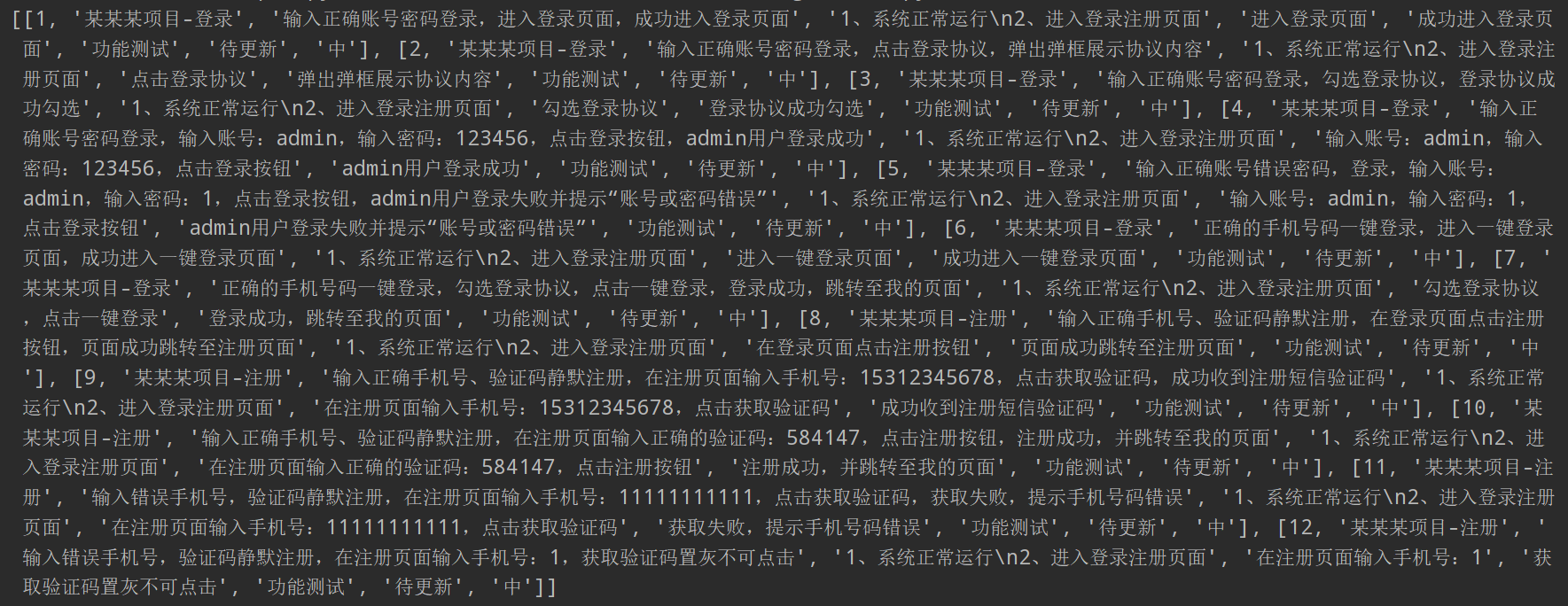``def change_case(listcase,top_term='',case_type='功能测试',case_state='待更新',case_grade='中'):    """    Excel中用例标题分为如下9个（tapd上的上传标准）：    ID用例目录用例名称前置条件用例步骤预期结果用例类型用例状态用例等级    ID：自增    用例目录：项目名称-模块名称 例如：某某某项目-登录模块    用例名称：提取传入数据的三级标题+四级标题+五级标题    前置条件：默认为空，可自己填写通用数据    用例步骤：提取数据的四级标题    预期结果：提取数据的五级标题    用例类型：默认值为功能测试，可填其他值为：性能测试，安全性测试    用例状态：默认值为待更新，可填其他值为：正常，已废弃    用例登记：默认值为中，可填其他值为：高，低    :param data_list: 传入已从xmind分解成的list数据    :param top_term: 前置条件    :param case_type: 用例类型    :param case_state: 用例状态    :param case_grade: 用例等级    :return: 返回以一条用例为一个list的数据，例如[[用例1],[用例2],[用例3]]    """    total_case=[]#总的用例格式的list    case_id=1    for data in listcase: case_list=[]#每一条用例的list data_sp=data.split('_') case_list.append(case_id) case_list.append(data_sp+"-"+data_sp) case_list.append(data_sp+'，'+data_sp+'，'+data_sp) case_list.append(top_term) case_list.append(data_sp) case_list.append(data_sp) case_list.append(case_type) case_list.append(case_state) case_list.append(case_grade) total_case.append(case_list) case_id+=1    return total_case``

## 4、数据写入Excel中

``def write_excel_case(total_case,save_path):"""    把解析的数据写入Excel中    :param total_case: 解析完成的数据    :param save_path: 文件保存路径    :return:     """    wb=Workbook()    ws=wb.active    ws['A1']='ID'    ws['B1']='用例目录'    ws['C1']='用例名称'    ws['D1']='前置条件'    ws['E1']='用例步骤'    ws['F1']='预期结果'    ws['G1']='用例类型'    ws['H1']='用例状态'    ws['I1']='用例等级'    i=1    for case in total_case: ws['A{}'.format(i + 1)] = case ws['B{}'.format(i + 1)] = case ws['C{}'.format(i + 1)] = case ws['D{}'.format(i + 1)] = case ws['E{}'.format(i + 1)] = case ws['F{}'.format(i + 1)] = case ws['G{}'.format(i + 1)] = case ws['H{}'.format(i + 1)] = case ws['I{}'.format(i + 1)] = case i+=1    column_list = ['A', 'B', 'C', 'D', 'E', 'F', 'G', 'H', 'I']    cell_list = ['A1', 'B1', 'C1', 'D1', 'E1', 'F1', 'G1', 'H1', 'I1']    #设置表头字体    font=Font(name='宋体',bold=True)    for cel in cell_list: cell=ws[cel] cell.font=font    # 设置列的表格居中    alignment = Alignment(horizontal='center',wrapText=True)    for col_name in column_list: col_list = ws[col_name] for col in col_list:     col.alignment = alignment    #设置列的宽度    ws.column_dimensions['B'].width = 20    ws.column_dimensions['C'].width = 60    ws.column_dimensions['D'].width = 30    ws.column_dimensions['E'].width = 30    ws.column_dimensions['F'].width = 30    ws.column_dimensions['G'].width = 15    ws.column_dimensions['H'].width = 15    ws.column_dimensions['I'].width = 15    #设置行的高度    for x in range(2,ws.max_row+1): ws.row_dimensions[x].height = 40    wb.save(save_path)``

## 5、运行入口

``def run_main(xmind_file_path,save_path,top_term='',case_type='功能测试',case_state='待更新',case_grade='中'):    """    :param xmind_file_path: xmind文件路径    :param save_path: 文件保存路径    :param top_term: 前置条件    :param case_type: 用例类型    :param case_state: 用例状态    :param case_grade: 用例等级    :return:    """    title,data_list=read_xmind_data(xmind_file_path)    listcase=xmind_to_caselist(data_list,title,[])    total_case = change_case(listcase, top_term=top_term,case_type=case_type,case_state=case_state,case_grade=case_grade)    write_excel_case(total_case,save_path)``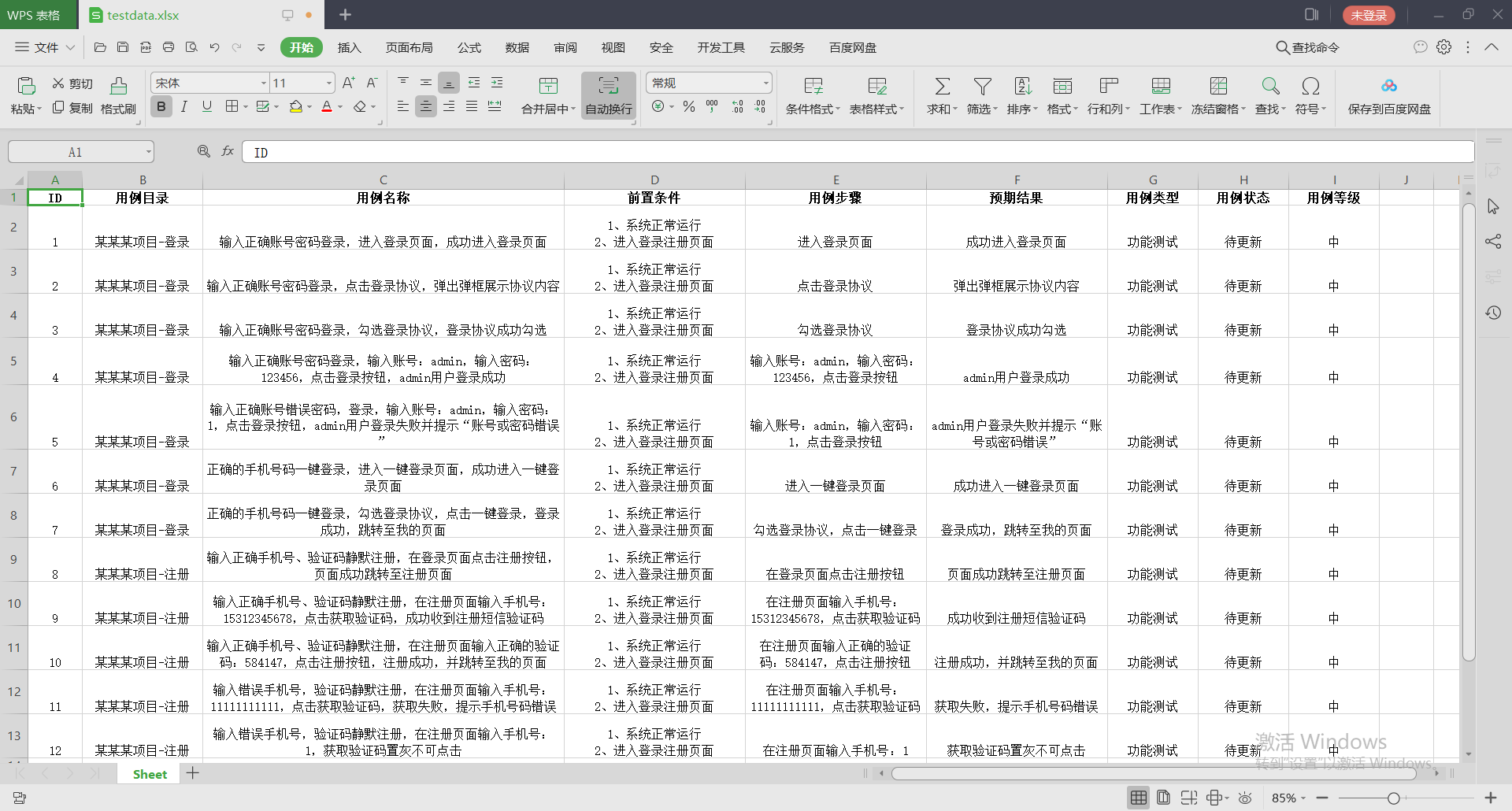# 四、总结开发者涨薪指南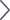48位大咖的思考法则、工作方式、逻辑体系小故事网